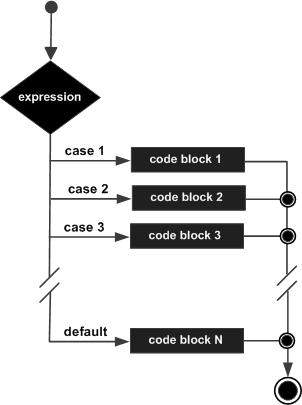# C++ switch statement

Advertisements

A switch statement allows a variable to be tested for equality against a list of values. Each value is called a case, and the variable being switched on is checked for each case.

## Syntax

The syntax for a switch statement in C++ is as follows −

```switch(expression) {
case constant-expression  :
statement(s);
break; //optional
case constant-expression  :
statement(s);
break; //optional

// you can have any number of case statements.
default : //Optional
statement(s);
}
```

The following rules apply to a switch statement −

• The expression used in a switch statement must have an integral or enumerated type, or be of a class type in which the class has a single conversion function to an integral or enumerated type.

• You can have any number of case statements within a switch. Each case is followed by the value to be compared to and a colon.

• The constant-expression for a case must be the same data type as the variable in the switch, and it must be a constant or a literal.

• When the variable being switched on is equal to a case, the statements following that case will execute until a break statement is reached.

• When a break statement is reached, the switch terminates, and the flow of control jumps to the next line following the switch statement.

• Not every case needs to contain a break. If no break appears, the flow of control will fall through to subsequent cases until a break is reached.

• A switch statement can have an optional default case, which must appear at the end of the switch. The default case can be used for performing a task when none of the cases is true. No break is needed in the default case.

## Flow Diagram## Example

```#include <iostream>
using namespace std;

int main () {
// local variable declaration:
char grade = 'D';

switch(grade) {
case 'A' :
cout << "Excellent!" << endl;
break;
case 'B' :
case 'C' :
cout << "Well done" << endl;
break;
case 'D' :
cout << "You passed" << endl;
break;
case 'F' :
cout << "Better try again" << endl;
break;
default :
cout << "Invalid grade" << endl;
}
cout << "Your grade is " << grade << endl;

return 0;
}
```

This would produce the following result −

```You passed
Your grade is D
```
cpp_decision_making.htm
Advertisements